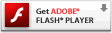# Interactive Generative Art Series – 05 – wild anchorWhile in the previous step (Generative Art 04 Using a target other than the mouse) in this generative actionscript art tutorial series it was cool to see everything move on it’s very own, it seemed a bit slow or fake, or maybe just plain uninteresting. Tinkering with the color, I thought if we set a minimum and maximum value for each red green and blur we could control the colors a bit more and still let them be generative. Plus I wanted the anchor to move a bit more and thus paint the curves and lines in a more interesting fashion. To do this we ramp up the range of the change rate of the anchor velocity. I really enjoy this example because it is faster, so we get more of those sweeping arcs, but also when the anchor slows down we get some very delicate curves and twists. With just a couple changes from the last example (which frankly seemed a bit chaotic), now I’m starting to see for the first time how to set some controls in the code which will lead to a visually appealing and still randomly generative result.For some reason I find it gratifying that the final swf is still a mere 2kb and change. Perhaps all this current focus on HD and 3D gives us the sense that to be good it needs to have a large footprint. Sometimes the magic or value is in how much you can accomplish with less (less is more)

### 05 Wild Anchor, play here

Please visit the blog article to view this interactive flash content. Flash plug-in required:### actionscript source code

[cc lang=”actionscript”]
var ball:Sprite = new Sprite();
ball.graphics.beginFill(0x000000, .5);
ball.graphics.drawCircle(0, 0, 5);
ball.graphics.endFill();

var anchor:Sprite = new Sprite();
anchor.graphics.beginFill(0x333333, .5);
anchor.graphics.drawCircle(0, 0, 12);
anchor.graphics.endFill();

var div:Number = .1;
var line_max_width:Number = 48;
var line_min_width:Number = 1;
var line_width:Number = randomRange(line_min_width, line_max_width);
var line_width_velocity:Number = 0;
var dampen:Number = 0.95;

var ballax:Number = 0;
var ballay:Number = 0;
var oldx:Number = ball.x;
var oldy:Number = ball.y;

var anchorvx:Number = 0;
var anchorvy:Number = 0;

anchor.x = stage.stageWidth/2;
anchor.y = stage.stageHeight/2;

var colors:Object = new Object();
colors.r = 255;
colors.g = 255;
colors.b = 255;
colors.rv = 0;
colors.gv = 0;
colors.bv = 0;
colors.rmin = 150; //0
colors.rmax = 250; //100
colors.gmin = 0; //100
colors.gmax = 150; //200
colors.bmin = 0; //150
colors.bmax = 100; //250
colors.rate_of_change = 12;
var color_first:Number = 0xFFFFFF;
var color_second:Number = rgb2hex(colors.r, colors.g, colors.b);

function loop () {

oldx = ball.x;
oldy = ball.y;

anchorvx += randomRangeAxis(10);
anchorvy += randomRangeAxis(10);

anchor.x += anchorvx;
anchor.y += anchorvy;

anchorvx *= dampen;
anchorvy *= dampen;

if(anchor.x > stage.stageWidth) {
anchor.x = 0 – anchor.width;
}
else if(anchor.x < 0 – anchor.width) { anchor.x = stage.stageWidth; } if(anchor.y > stage.stageHeight) {
anchor.y = 0 – anchor.height;
}
else if(anchor.y < 0 – anchor.height) { anchor.y = stage.stageHeight; } ball.x -= ballax = (ballax + (ball.x – anchor.x) * div) * .9; ball.y -= ballay = (ballay + (ball.y – anchor.y) * div) * .9; line_width_velocity += randomRangeAxis(1); line_width += line_width_velocity; line_width_velocity *= dampen; if(line_width > line_max_width) {
line_width = line_max_width;
line_width_velocity = 0;
}
else if (line_width < line_min_width) {
line_width = line_min_width;
line_width_velocity = 0;
}

color_step();
color_first = color_second;
color_second = rgb2hex(colors.r, colors.g, colors.b);

var dx:Number = ball.x – oldx;
var dy:Number = ball.y – oldy;
this.graphics.lineStyle(line_width);
this.graphics.lineTo(ball.x, ball.y);
}

setInterval(loop, 1000/30);
function rgb2hex(r:Number, g:Number, b:Number):Number {
return(r<<16 | g<<8 | b); } function color_step(){ colors.rv += randomRangeAxis(colors.rate_of_change); colors.r += colors.rv; colors.rv *= dampen; if (colors.r > colors.rmax) {
colors.r = colors.rmax;
} else if (colors.r < colors.rmin){ colors.r = colors.rmin; } colors.gv += randomRangeAxis(colors.rate_of_change); colors.g += colors.gv; colors.gv *= dampen; if (colors.g > colors.gmax) {
colors.g = colors.gmax;
} else if (colors.g < colors.gmin){ colors.g = colors.gmin; } colors.bv += randomRangeAxis(colors.rate_of_change); colors.b += colors.bv; colors.bv *= dampen; if (colors.b > colors.bmax) {
colors.b = colors.bmax;
} else if (colors.b < colors.bmin){
colors.b = colors.bmin;
}
}
//random number between min and max
function randomRange(max:Number, min:Number = 0):Number {
return Math.random() * (max – min) + min;
}
//random number range centered at 0 with the specified max, randomRange(-max, max)
function randomRangeAxis(max:Number):Number {
return Math.random() * (max * 2) – max;
}

[/cc]